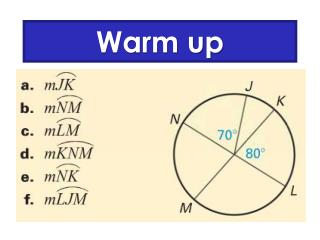DownloadDownload PresentationWarm up

# Warm up

Download Presentation## Warm up

- - - - - - - - - - - - - - - - - - - - - - - - - - - E N D - - - - - - - - - - - - - - - - - - - - - - - - - - -
##### Presentation Transcript

1. Warm up

2. Review HW

3. Wheel of Formulas!!

4. Central Angle Angle = Arc

5. Inscribed Angle • Angle where the vertex in ON the circle

6. Inscribed Angle

7. 160 The arc is twice as big as the angle!! 80

8. Find the value of x and y. 120  x  y 

9. J K Q S M Examples 1. If mJK= 80 and JMK = 2x – 4, find x. x = 22 2. If mMKS = 56, find m MS. 112 

10. Find the measure of DOG and DIG D 72˚ G If two inscribed angles intercept the same arc, then they are congruent. O I

11. If all the vertices of a polygon touch the edge of the circle, the polygon is INSCRIBED and the circle is CIRCUMSCRIBED.

12. Quadrilateral inscribed in a circle: opposite angles are SUPPLEMENTARY B A D C

13. If a right triangle is inscribed in a circle then the hypotenuse is the diameter of the circle. diameter

14. Q D 3 J T 4 U Example 3 In J, m3 = 5x and m4 = 2x + 9. Find the value of x. x = 3

15. Example 4 In K, GH is a diameter and mGNH = 4x – 14. Find the value of x. 4x – 14 = 90 H K x = 26 N G Bonus: What type of triangle is this? Why?

16. Example 5 Find y and z. z 110 110 + y =180 y y = 70 85 z + 85 = 180 z = 95

17. Homework WS Inscribed Angles Choose even or odd. Everyone does the last problem.